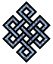#fail2ban bad ip database: ip 82.223.32.38

### | ip database | live view | stats | report | help | api key:

 ip: 82.223.32.38 hostname: 82.223.32.38 country:[ES] Spain first reported: 25.09.2018 02:15.54 GMT+0200 last reported: 12.03.2019 14:09.53 GMT+0200 time period: 168d 12h 53m 59s total reports: 4 reported by: 3 host(s) filter(s): ssh (3) ssh (1) tor exit node no badips.com db Lookup## port scan of '82.223.32.38':

[-hide]
```# Nmap 6.40 scan initiated Tue Sep 25 02:16:02 2018 as: /usr/bin/nmap -sU -sS -O 82.223.32.38
Nmap scan report for 82.223.32.38
Host is up (0.040s latency).
Not shown: 1000 open|filtered ports, 995 filtered ports
PORT     STATE  SERVICE
22/tcp   open   ssh
80/tcp   open   http
443/tcp  closed https
8443/tcp closed https-alt
No exact OS matches for host (If you know what OS is running on it, see http://nmap.org/submit/ ).
TCP/IP fingerprint:
OS:SCAN(V=6.40%E=4%D=9/25%OT=22%CT=443%CU=%PV=N%G=Y%TM=5BA97E6E%P=x86_64-pc
OS:-linux-gnu)SEQ(SP=106%GCD=1%ISR=109%TI=Z%CI=I%TS=8)OPS(O1=M5B4ST11NW7%O2
OS:=M5B4ST11NW7%O3=M5B4NNT11NW7%O4=M5B4ST11NW7%O5=M5B4ST11NW7%O6=M5B4ST11)W
OS:IN(W1=7120%W2=7120%W3=7120%W4=7120%W5=7120%W6=7120)ECN(R=Y%DF=Y%TG=40%W=
OS:7210%O=M5B4NNSNW7%CC=Y%Q=)T1(R=Y%DF=Y%TG=40%S=O%A=S+%F=AS%RD=0%Q=)T2(R=N
OS:)T3(R=N)T4(R=Y%DF=Y%TG=40%W=0%S=A%A=Z%F=R%O=%RD=0%Q=)T5(R=Y%DF=Y%TG=40%W
OS:=0%S=Z%A=S+%F=AR%O=%RD=0%Q=)T6(R=Y%DF=Y%TG=40%W=0%S=A%A=Z%F=R%O=%RD=0%Q=
OS:)T7(R=N)U1(R=N)IE(R=Y%DFI=N%TG=40%CD=S)

OS detection performed. Please report any incorrect results at http://nmap.org/submit/ .
# Nmap done at Tue Sep 25 02:16:46 2018 -- 1 IP address (1 host up) scanned in 44.66 seconds
```
```Σ = 88 | Δt = 0.012892007827759s
```